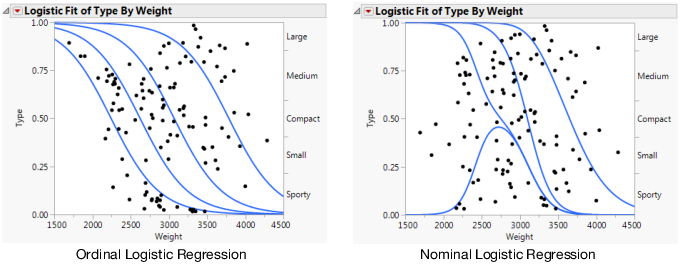Basic Analysis > Logistic Analysis
Publication date: 05/24/2021

# Logistic Analysis

##### Examine Relationships between a Categorical Y and a Continuous X Variable

Use the Logistic platform to fit a logistic regression model to a categorical response with a continuous x predictor. Options include a ROC curve, lift curve, and odds ratio estimates. The fitted model estimates probabilities for each x value. The Logistic platform is the nominal or ordinal by continuous personality of the Fit Y by X platform. There is a distinction between nominal and ordinal responses on this platform:

Nominal logistic regression estimates a set of curves to partition the probability among the responses.

Ordinal logistic regression models the probability of being less than or equal to a given response. This has the effect of estimating a single logistic curve, which is shifted horizontally to produce probabilities for the ordered categories. This model is less complex and is recommended for ordered responses.

Figure 8.1 Examples of Logistic Regression